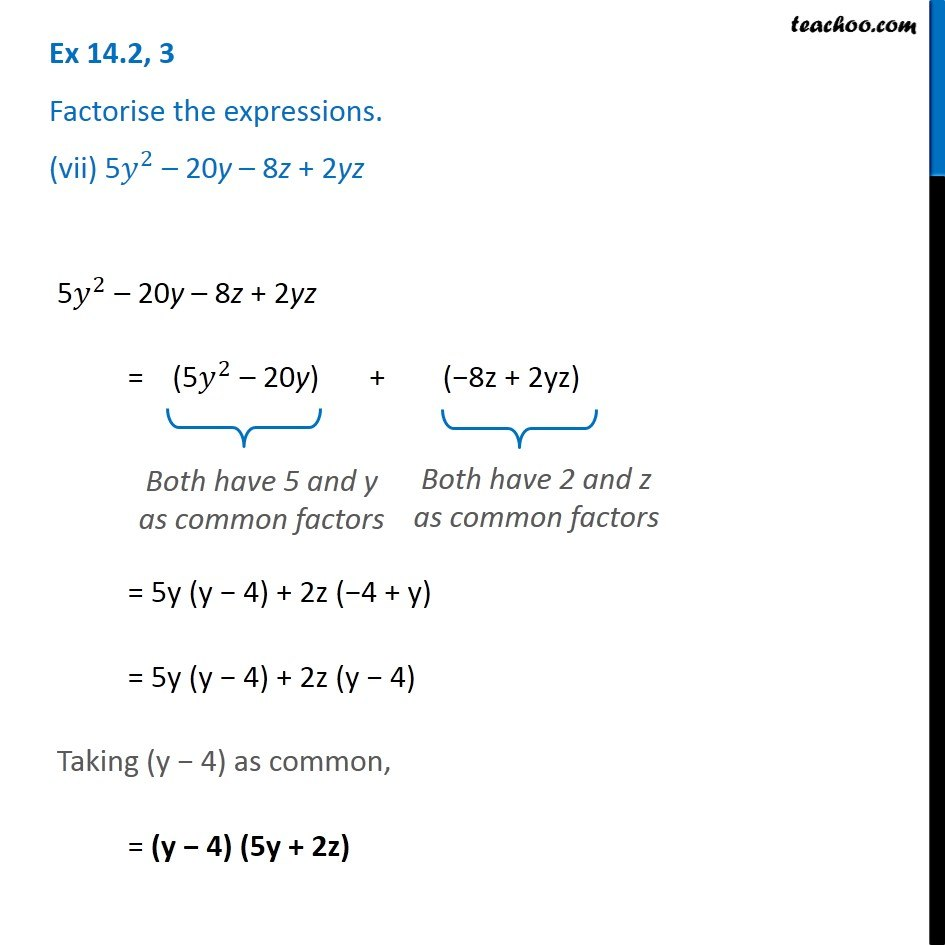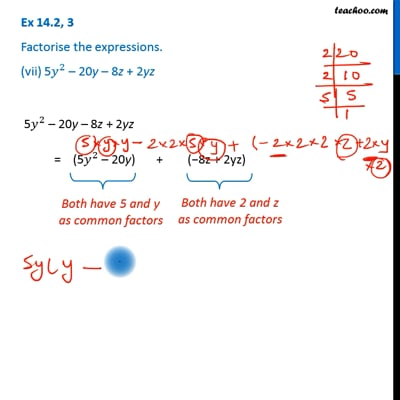Ex 14.2

Chapter 14 Class 8 Factorisation
Serial order wiseThis video is only available for Teachoo black usersThis video is only available for Teachoo black users

Get live Maths 1-on-1 Classs - Class 6 to 12

### Transcript

Ex 14.2, 3 Factorise the expressions. (vii) 5𝑦^2 – 20y – 8z + 2yz 5𝑦^2 – 20y – 8z + 2yz = (5𝑦^2 – 20y) + (−8z + 2yz) Both have 5 and y as common factors Both have 2 and z as common factors Both have 2 and z as common factors = 5y (y − 4) + 2z (−4 + y) = 5y (y − 4) + 2z (y − 4) Taking (y − 4) as common, = (y − 4) (5y + 2z)